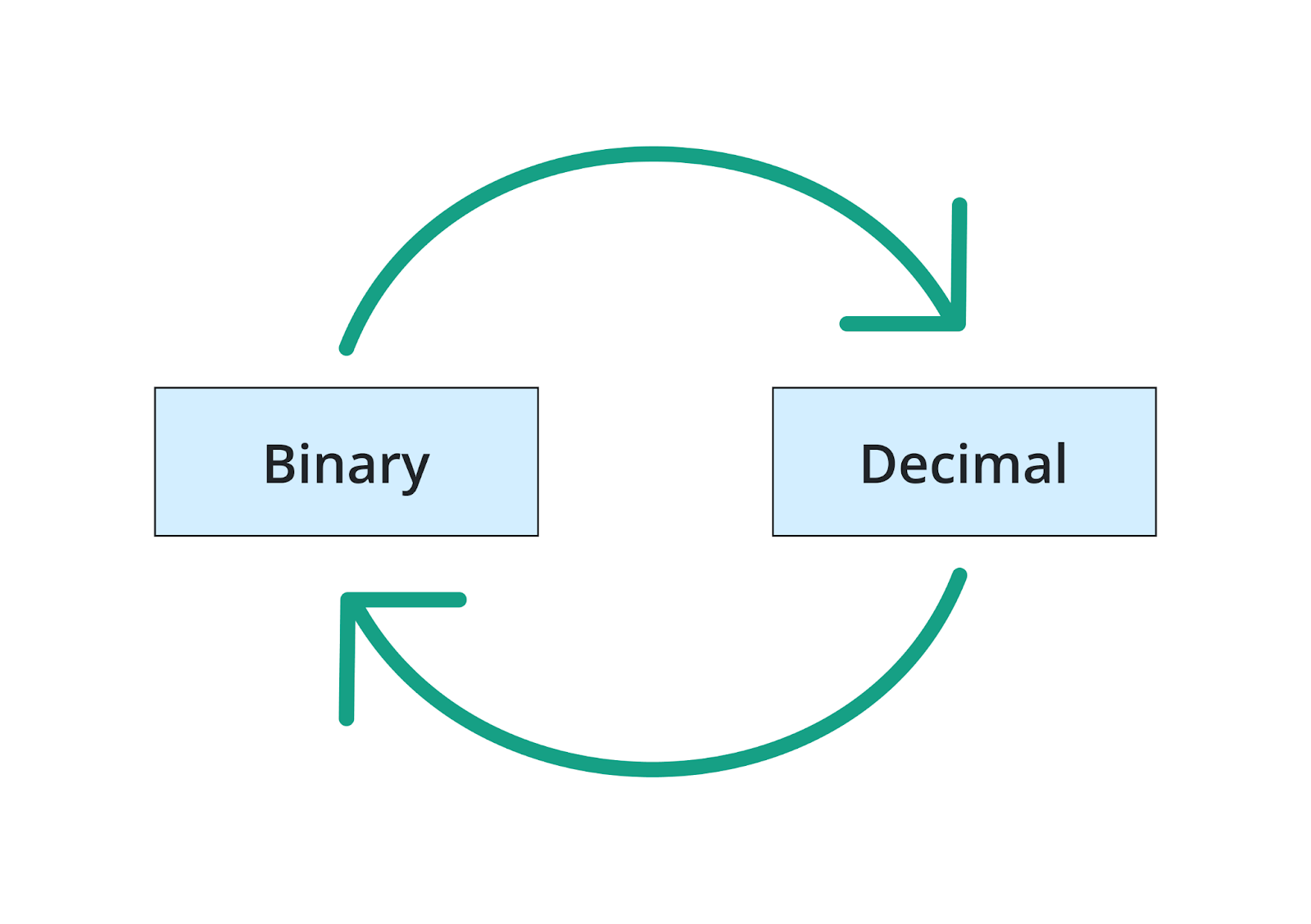# Binary to Decimal Converter

Enter the binary value in the input box and hit the "calculate" button to convert your binary numbers into their corresponding decimal and hex numbers using this Binary to Decimal Converter.

Give Us Feedback

## Binary to Decimal Converter

Binary to Decimal Converter is used in computers and mathematics to change values from one system to another.

The calculator provides complete steps of conversion along with the hex and decimal number representations. It is fast and easy.

## What is binary to decimal conversion?

Although binary numbers are very important in computer sciences, they have their limitations. Also, humans have a hard time decoding information from them.

This is why binary to decimal conversion is as important as a decimal to binary conversion. It is changing digits from one system into the digits that are accepted into the other system.## How to convert binary numbers to decimals?

The conversion from binary to decimal is relatively simpler than the other way round. Read on to learn the steps involved.

1. Number the position of each digit.
2. Multiply each digit with 2 raised to power one less than its position.
3. Now, add all the values.
4. The number is in the decimal system.

The binary translator to numbers is still a recommended shortcut. Let’s see binary to decimal conversion examples.

Example 1:

What is the decimal number for 01010110?

Solution:
Step 1: Write the position of each digit in front of it.

0 (8)
1 (7)
0 (6)
1 (5)
0 (4)
1 (3)
1 (2)
0 (1)

Step 2: Subtract one from each position and raise it as the power of 2

0 (27
1 (26
0 (25
1 (24
0 (23
1 (22
1 (21
0 (20)

Step 3: Now, multiply these numbers.

0 (27) = 0 x 128 = 0
1 (26) = 1 x 64   = 64
0 (25) = 0 x 32   = 0
1 (24) = 1 x 16   = 16
0 (23) = 0 x 8     = 0
1 (22) = 1 x 4     = 4
1 (21) = 1 x 2     = 2
0 (20) = 0 x 1     = 0

= 0 + 64 + 0 + 16 + 0 + 4 + 2 + 0
= 86

Example 2:
Convert the binary value 110011 into a decimal value.

Solution:
Step 1: Write the powers of two as done in the previous example.

= `1 (25) + 1 (24) + 0 (23) + 0 (22) + 1 (21) + 1 (20)`

Step 2: Multiply and add to find the binary value.

= 1 x 32 + 1 x 16 + 0 x 8 + 0 x 4 + 1 x 2 + 1 x 1
= 32 + 16 + 0 + 0 + 2 +  1
= 51

### Math Tools